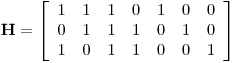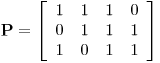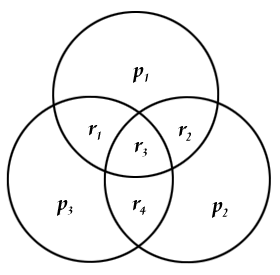# Hex, Bugs and More Physics | Emre S. Tasci

a blog about physics, computation, computational physics and materials…

## (7,4) Hamming Code

### November 23, 2007 Posted by Emre S. Tasci

The (7,4) Hamming Code is a system that is used for sending bits of block length 4 (16 symbols) with redundancy resulting in transmission of blocks of 7 bits length. The first 4 bits of the transmitted information contains the original source bits and the last three contains the parity-check bits for {1,2,3}, {2,3,4} and {1,3,4} bits.

It has a transmission rate of 4/7 ~ 0.57 . Designate the source block as s, the transmission operator as G so that transmitted block t is t = GTs and parity check operator is designated as H where:This could be more obvious if we investigate the GT and keeping in mind that, the first 4 "transformed" bits stay the same with 3 added to the end for parity check. So our parity reporter operator P would be:As it calculates the parities for bits, also its Modulo wrt 2 must be included. Since we’re calculating the parities for {1,2,3}, {2,3,4} and {1,3,4} bit-groups, the values of the P are easily understandable. Now, we will join the Identity Matrix of order 4 (for directly copying the first 4 values) with the P operator to construct the GT transformation (encoder) operator:and H is actually nothing but [-P I3]. It can be seen that HGTs will always result {0,0,0} in a parity check, meaning that all three parities have passed the test. So, where is the problem?

Noise: Noise is the operator that flips the values of bits randomly with an occuring probability f. Suppose that we have a source signal block of 4 bits length: {s1, s2, s3, s4}. I encode this information with calculating the parities and adding them to the end, thus coming up with a block of 7 bits length and call this block as t (for transmitted):

t=GTs (supposing t and s are given as column vectors. Otherwise, t=sG)

Now I send this packet but on the road to the receiver, the noise changes it and so, the received message, r, is the transmitted message (t) AND the noise (n):

r=t+s

Since I have got the parity information part (which may also have been affected by the noise), I compare the received data’s first 4 values with the parity information stored in the last 3 values. A very practical way of doing this is using 3 intersecting circles and placing them as:with r1-r4 representing the first values (ie, actual data) received and p1-p3 being the parity information (the last 3 values). So we place the values to their corresponding locations and easily can check if the parity matches to the related values. We can have 8 different situations at this moment. Let’s denote the z=Hr vector as the parity check result. z={0,0,0} if all three parity information agrees with the calculated ones, z={1,0,0} if only p1 is different from the calculated parity of {r1,r2,r3}, z={1,0,1} if p1 AND p3 are different from their corresponding calculated parities and so on. If there is only 1 error (ie, only one "1" and two "0") in the parity check, then it means that, the noise affected only the parity information, not the data encoded (ie, the first 4 values), so the corrupted data can be fixed by simply switching the faulty parity. If there are 2 parity check errors, this time, the value on the intersection of the corresponding parities (ie, r1 for p1&p3, r2 for p1&p2 and r4 for p2&p3,) must be switched. If z={1,1,1} then r3 should be switched.

Let’s introduce some mathematica operations and then tackle with some examples. You can just copy and paste them since there are no additional libraries required:

mGT = {{1,0,0,0},{0,1,0,0},{0,0,1,0},{0,0,0,1},{1,1,1,0},{0,1,1,1},{1,0,1,1}};
mH = {{1,1,1,0,1,0,0},{0,1,1,1,0,1,0},{1,0,1,1,0,0,1}};

(mS = {{1},{1},{1},{0}});
Mod[(mGTS=mGT . mS),2] // MatrixForm
mN ={0,0,0,0,0,0,1};
(mR = Mod[mGTS+mN,2])  // MatrixForm
mZ = Mod[mH . mR,2];
Flatten[mZ]

The first two lines define GT and H and from here, you can check a very important property being:

Mod[mH.mGT,2] // MatrixForm

equal to 0.

We introduce the 4bit data to be transmitted as mS which we had referred as s through out the text. Then we encode the data to be send with the parity info encoded as mGTS (t) add a noise mN (n) to it assuming it is corrupted in the transmission process and the data the other side receives is mR (r) consisting of the data transmitted AND the noise acted on it. mZ is the parity check result (z) (I’ve "Flatten"ed it for a better view). In the operations above, the "Mod" operator is frequently used because of our universe being a mod2 universe (2=0, 3=1, 4=0,… just 0s and 1s actually!).

So, in this example, we had s={1,1,1,0}, and this was encoded as t={1,1,1,0,1,0,0} and on its way to the happy-ending, a noise corrupted it and switched its 3rd parity information from 0 to 1. As a result of this, the recipient got its data as r={1,1,1,0,1,0,1}. But since our recipient is not that dull, a parity check is immediately applied and it was seen that z={0,0,1} meaning that, there is something wrong with the 3rd parity information, and nothing wrong with the information received at all!

Now, let’s apply all the possible noises given that only one value can be switched at a time (ie, only one 1 in the n vector with the remaining all being 0s).

For[i = 1, i <= 7, i++,
mN = {0, 0, 0, 0, 0, 0, 0} ;
mN = ReplacePart[mN, 1, i];
mR = Mod[mGTS + mN, 2];
mZ = Mod[mH . mR, 2];
Print[mN, "\t", Flatten[mZ]];
];

After this, if everything went right, you’ll see the output something like this:

{1,0,0,0,0,0,0}   {1,0,1}
{0,1,0,0,0,0,0}   {1,1,0}
{0,0,1,0,0,0,0}   {1,1,1}
{0,0,0,1,0,0,0}   {0,1,1}
{0,0,0,0,1,0,0}   {1,0,0}
{0,0,0,0,0,1,0}   {0,1,0}
{0,0,0,0,0,0,1}   {0,0,1}

So we know exactly what went wrong at are able to correct it… Everything seems so perfect, so there must be a catch…

There is no decoding error as long as there is only 1 corruption per data block!

As the corruption number increases, there is no way of achieving the original data send with precise accuracy. Suppose that we re-send our previous signal s={1,1,1,0} which yields t={1,1,1,0,1,0,0} but this time the noise associated with the signal is n={0,1,0,0,0,0,1} so, the received signal is r={1,0,1,0,1,0,1}. When we apply the partition check, we have z={1,1,1}, which means that, there is a problem with the 3rd value and we deduce that the original message transmitted was t={1,0,0,0,1,0,1} which passes the parity check tests with success alas a wrongly decoded signal!

The probability of block error, pB is the probability that there will at least be one decoded bit that will not match the transmitted bit. A little attention must be paid about this statement. It emphasizes the decoded bit part, not the received bit. So, in our case of (4,7) Hamming Code, there won’t be a block error as long as there is only one corrupted bit in the received bit because we are able to construct the transmitted bits with certainty in that case. Only if we have two or more bits corrupted, we won’t be able to revive the original signal, so, pB scales as O(f2).

References : I have heavily benefitted from David MacKay’s resourceful book Information Theory, Inference, and Learning Algorithms which is available for free for viewing on screen.Information Theory, Inference, and Learning Algorithms by David MacKay

### One Response to “(7,4) Hamming Code”

1. Hex, Bugs and More Physics | Emre S. Tasci » Blog Archive » Bayesian Inference - An introduction with an example Says:

[…] that Jo (of Example 2.3 in MacKay’s previously mentioned book), decided to take a test to see whether she’s pregnant or not. She applies a test that […]